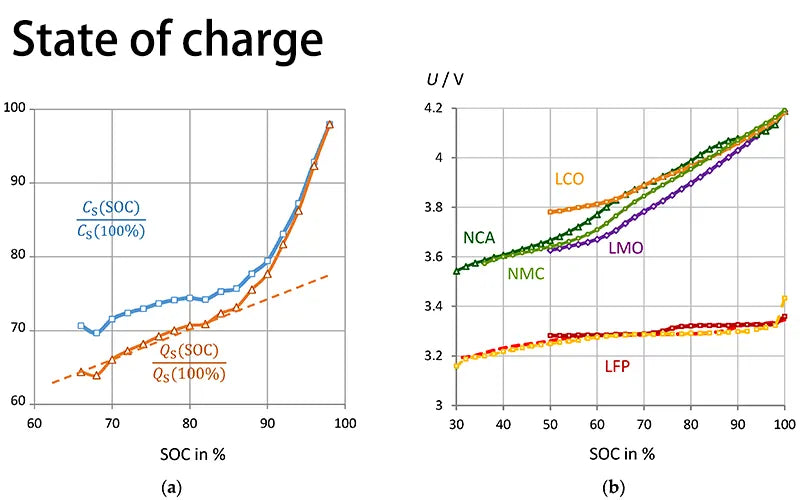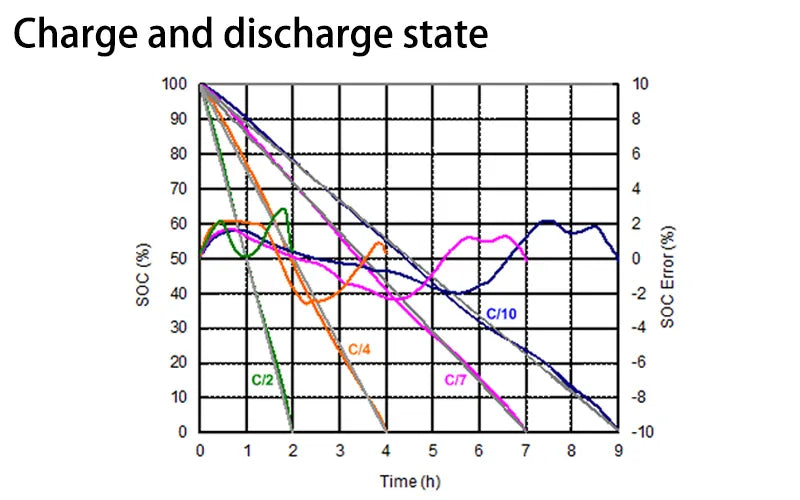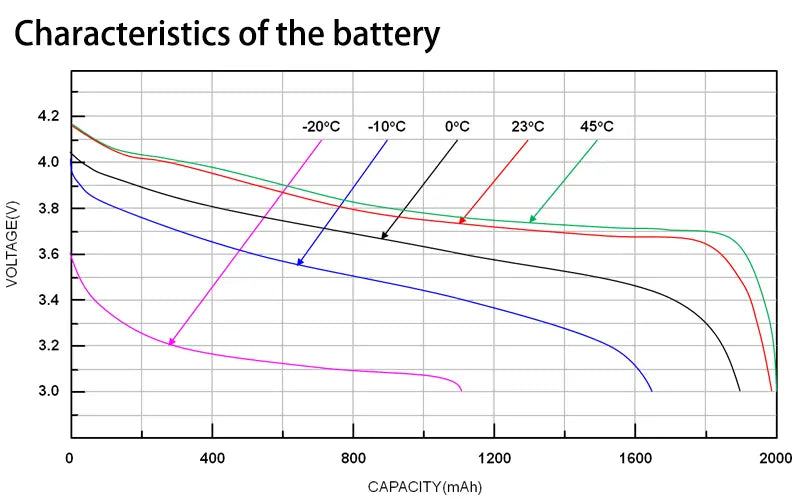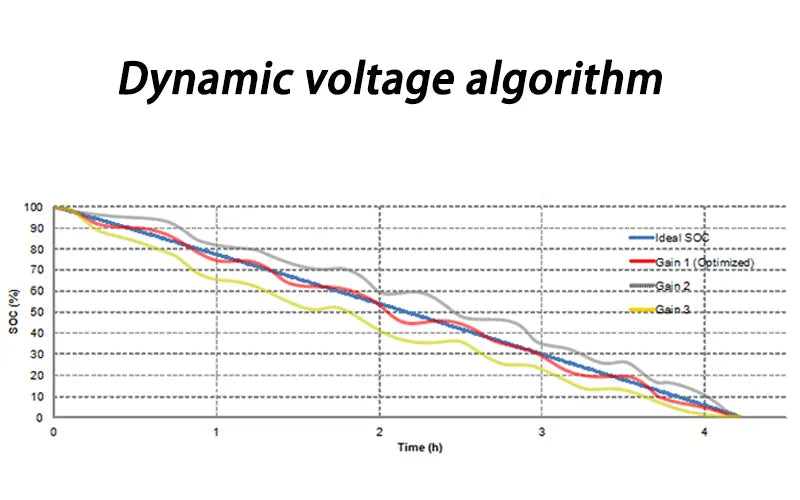## 1. Introduction of lithium-ion battery

A lithium-ion battery is a secondary battery (rechargeable battery) that mainly relies on lithium ions to move between the anode and cathode to function.

During the charge-discharge process, li+ is embedded back and forth and unleaved. When in charging, li+ is deinterleaved from the anode and embedded in the cathode through the electrolyte, and the cathode is in a lithium-rich state. The opposite is true when discharging.

Portable devices like mobile phones and laptops use lithium-ion batteries, especially lifepo4 batteries. Additionally, batteries generally use lithium-containing materials, which are representative of modern high-performance batteries, as electrodes.

## 2. State of charge

The state of charge can be defined as the state of available energy in the battery, usually expressed as a percentage. Because the available electrical energy will vary according to the charge and discharge current, temperature and aging phenomenon, the definition of charge state is also divided into two types: absolute state-of-charge( ASOC) and relative state-of-charge ( RSOC).

The typical range for the relative state of charge is 0% - 100%, while the battery is 100% fully charged and 0% when fully discharged.

The absolute state of charge is a reference value calculated based on the designed fixed capacity value when the battery is manufactured. The absolute state of charge of a new fully charged battery is 100%. Even if the aging battery is fully charged, it cannot reach 100% under different charging and discharging conditions.The relationship between voltage and battery capacity at different discharge rates. The higher the discharge rate, the lower the battery capacity. When the temperature is low, the battery capacity is also reduced.

## 3. Charge and discharge state

● Max charging voltage

The maximum charging voltage and the chemical composition of the battery are related to the characteristics. The charging voltage of lithium batteries is usually 4.2V and 4.35V, and the voltage value will be different if the cathode and anode materials are different.

The battery voltage is one of the important indicators to measure the discharge performance. Take the car battery voltage as example, on the circumstance of no-load, the normal voltage of the car battery is about 13V while the load voltage often exceeds 11V. It will be difficult to start when the voltage is lower. Hence the normal voltage of the motor cells should be higher.

● Fully charged

When the difference between the battery voltage and the maximum charge voltage is less than 100mV, and the charging current is reduced to C/10, the battery is considered fully charged.The battery characteristics are different, and the full charging conditions are also different. When the battery voltage is equal to the maximum charging voltage and the charging current is reduced to C/10, the battery is considered fully charged.

● Mini discharging voltage

The minimum discharge voltage can be defined by the cut-off discharge voltage, which is usually the voltage at 0% charge. This voltage value is not a fixed value, but changes with load, temperature, aging or other factors.

● Fully discharge

When the battery voltage is less than or equal to the minimum discharge voltage, it can be called complete discharge.

## 4. Characteristics of the battery

● Charge-discharge rate

The charge-discharge rate is a representation of the charge-discharge current relative to the battery capacity. For example, if 1C is used to discharge for one hour, ideally, the battery will be completely discharged. Different charge/discharge rates result in different usable capacities. In general, the larger the charge-discharge rate, the smaller the usable capacity.

● Cycle life

The number of cycles is the number of times a battery has been fully charged and discharged, which can be estimated from the actual discharge capacity and design capacity.

The number of cycles is one whenever the accumulated discharge capacity is equal to the design capacity. Usually after 500 charge-discharge cycles, the fully charged battery capacity drops by 10% to 20%.● Self-discharge

The self-discharge of all batteries increases as the temperature rises. Self-discharge is basically not a manufacturing defect, but a characteristic of the battery itself. However, improper handling during the manufacturing process can also lead to an increase in self-discharge.

Generally, for every 10°C increase in battery temperature, the self-discharge rate doubles. The self-discharge capacity of lithium-ion batteries is about 1~2% per month, while that of various nickel batteries is 10~15% per month.

## 4. Introduction to battery fuel gauges

Battery management can be thought of as part of power management. In battery management, the fuel gauge is responsible for estimating battery capacity. Its basic functions are to monitor voltage, charge/discharge current, and battery temperature, and estimate battery soc (state of charge) and full charge capacity (FCC) .

There are two typical methods for estimating the state of charge of a battery: open circuit voltage (OCV) and coulombic metering. Another method is a dynamic voltage algorithm.

### ① Open circuit voltage method

The opencircuit voltage is assumed to be the battery terminal voltage when the battery rests for about 30 minutes. Depending on the load, temperature, and battery aging, the battery voltage curve will also be different.

Therefore, a fixed open circuit voltmeter cannot fully represent the state of charge; the state of charge cannot be estimated by looking at tables alone. In other words, if the load state is estimated only by looking up the table, the error will be large.

Under different loads during discharge, the difference in state of charge is also very large. So basically, the open-circuit voltage method is only suitable for systems with low requirements for state-of-charge accuracy, such as lead-acid batteries or uninterruptible power supplies used in automobiles.

### ② Coulomb metrology

Coulomb metrology operates by connecting a sense resistor along the battery's charge/discharge path. The ADC measures the voltage across the sense resistor and converts it into the current value at which the battery is charging or discharging.

A real-time counter (RTC) provides the time to integrate this current value to know how many coulombs are flowing. Coulomb metrology accurately calculates the real-time load state during loading or unloading.

With the load column counter and the discharge column counter, it can calculate residual capacity (RM) and full load capacity (FCC). The state of charge can also be calculated using residual capacity (RM) and full charge capacity (FCC), i.e. (SOC = RM / FCC). In addition, it can estimate the remaining time, such as power exhaustion (TTE) and full power (TTF).

## 5. Calculation of coulomb metrology

There are two main factors that contribute to the accuracy bias of coulomb measurement. The first is the accumulation of offset errors in current sensing and ADC measurement. Although the error of this measurement is relatively small with current technology, it will increase over time without a good way to eliminate it.

To eliminate accumulated errors, there are three possible time points in normal battery operation.

● End of charge (EOC)

An end-of-charge condition indicates that the battery is fully charged and the state of charge (SOC) should be 100%.● End of discharge (EOD)

The end-of-discharge condition indicates that the battery is fully discharged and the state of charge (SOC) should be 0%.

● Rest (Relax)

When the rest state is reached, the battery is not charged or discharged, and it remains in this state for a long time.

The second major factor contributing to coulombology accuracy bias is the total charge capacity (FCC) error, which is the difference between the value of the battery's design capacity and the battery's true full charge capacity. Full load capacity (FCC) is affected by temperature, aging, load, etc.

## 6. Dynamic voltage algorithm

The dynamic voltage algorithm fuel gauge calculates the state of charge of a lithium battery based only on the battery voltage. This method estimates the increment or decrease of the charge state based on the difference between the battery voltage and the open circuit voltage of the battery.

Dynamic voltage information can effectively simulate the behavior of lithium batteries to determine the state-of-charge SOC (%), but this method does not estimate battery capacity value (mAh).

In contrast to Coulometric fuel gauge solutions, dynamic voltage algorithm fuel gauges do not accumulate errors over time and current. In addition, only voltage information is used to estimate the battery's state of charge.Compared to coulomb metering fuel gauges, which usually cause inaccurate charge status due to current sensing errors and battery self-discharge, the dynamic voltage algorithm does not accumulate errors over time and current, which is a big advantage.

Because there is no information on charge/discharge currents, dynamic voltage algorithms have poor short-term accuracy and slower response times. In addition, it is also impossible to estimate the full charging capacity. However, it performs well in long-term accuracy because the battery voltage ultimately directly reflects its state of charge.

Related articles: